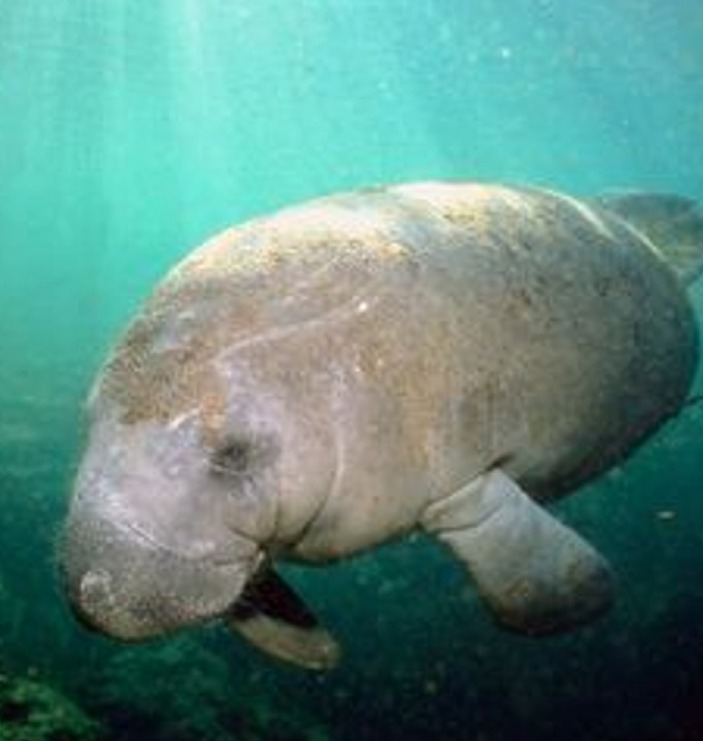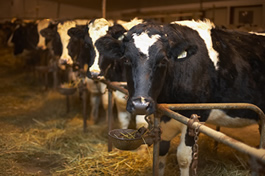Slope & Intercepts w/Linear Regressions Terms of Use    Contact Person: Donna RobertsDirections: Read carefully. Answers are rounded to nearest hundredths, unless stated otherwise.

 1.  Manatees are large, fully aquatic, highly intelligent, herbivorous marine mammals who must surface regularly for air. Marine biologists fear that the number of registered powerboats threatens the existence of manatees, as the animals are injured or killed by hit-and-runs and propellers.The chart at the right contains data collected from one location.

Prepare a linear regression model.
(Powerboats on x-axis, Manatees on y-axis.)

a)
What is the slope of the best fit linear regression equation?
 Choose: 0.98 -81.57 0.43
 Year Powerboat registration (in 1000s) Manatees Killed 2005 314 54 2006 351 70 2007 415 95 2008 469 125 2009 522 140 2010 574 167

b)
Which expression is an interpretation of the slope of the regression equation?
Choose:
 For each additional 100,000 powerboats registered, we can predict an additional 43 manatees will be killed. For each additional 100,000 powerboats registered, we can predict an additonal 98 manatees will be killed. For each additional 100,000 powerboats registered, we can predict an additional 8,157 manatess will be killed.

c)
Which is better for the manatees, positive residuals or negative residuals?
 Choose: positive residuals negative residuals

d) What does the model suggest regarding the future of the manatees?
 Choose: The increase in the number of powerboats is having no effect on the manatees. As the number of powerboats increases, the number of dead manatees will decrease. As the number of powerboats continues to increase, the number of dead manatees will increase.

2.  The table below shows the ages of eight cows (in years) and their milk production (in gallons) per week.

Prepare a linear regression model.
(Cow's Age on x-axis, Milk on y-axis.)

a)
Which type of correlation exists for this data?
 Choose: positive linear correlation negative linear correlation no linear correlation

b)
Which equation is the best fit linear regression equation for this data,
rounded to nearest hundredths?
 Choose: y = 1.07x + 40.79 y = -1.07x + 40.79 y = -0.95x + 41.20Cow's Age Gallons of Milk per Week 4 37.2 5 35.2 6 33.6 7 33.2 7 32.8 8 33.1 9 31.6 10 29.8

c)
What is the slope of the regression line telling us?
 Choose: For each additional year of age we can predict a weekly loss of 1.07 gallons of milk production. For each addional year of age, we can predict a weekly increase of 1.07 gallons of milk production. For each additional year of age, we can predict a weekly loss of 0.95 gallons of milk production.

d)
What is the y-intercept telling us?
 Choose: We can predict cows of age 3 to produce over 40 gallons of milk per week. We can predict cows of age 1 to produce over 40 gallons of milk per week. It is not telling us anything. Cows of age 0 do not produce milk.

3.  A survey of 16 shoppers at a mall was tallied to show their ages (in years) and the number of movies they saw over the past year.

Prepare a linear regression model.
(Age on x-axis, Movies on y-axis.)

a)
Which type of correlation exists for this data?
 Choose: positive correlation negative correlation no correlation

b)
What is the slope of the best fit linear regression equation?
 Choose: -1.94 -0.92 58.54

c) What is the y-intercept of the best fit linear regression equation?
 Choose: 30 -1.94 58.54

d) Using the regression equation, predict the number of movies that would be seen in one year by a 9 year old.
 Choose: 35 48 41Age (years) Number of Movies 18 24 24 11 17 24 24 16 26 9 25 8 17 28 19 20 16 30 20 16 18 25 22 20 22 12 25 12 21 13 23 14

e)
What is the slope of the regression line telling us?
 Choose: For each addional year of age, we can predict the person will see 0.92 additional movies. As people get older, they see more movies. For each additional year of age we can predict the person will see 1.94 fewer movies. As people get older, they see fewer movies. The slope is not telling us enough information to make a prediction.